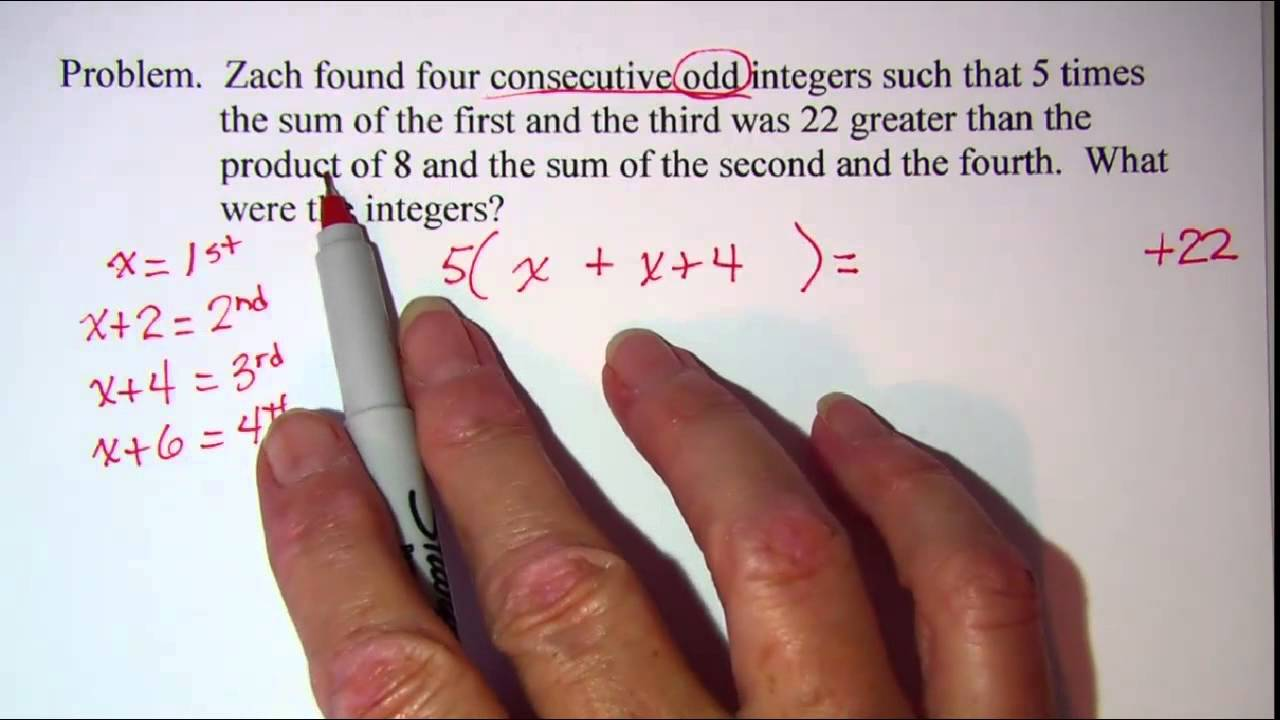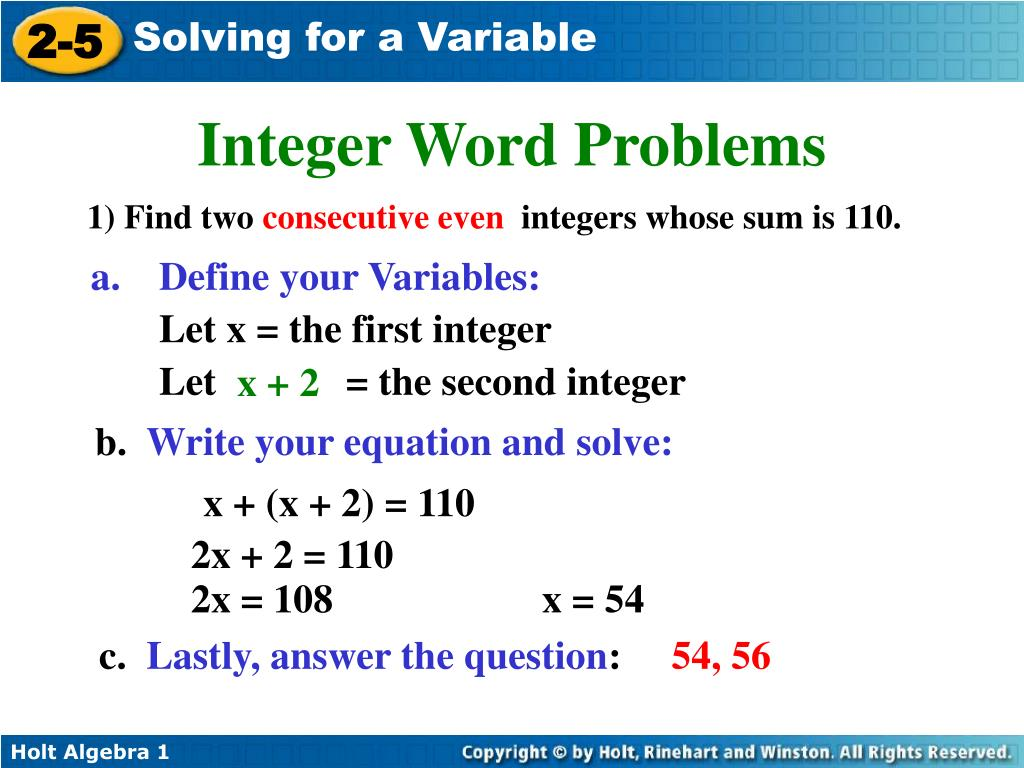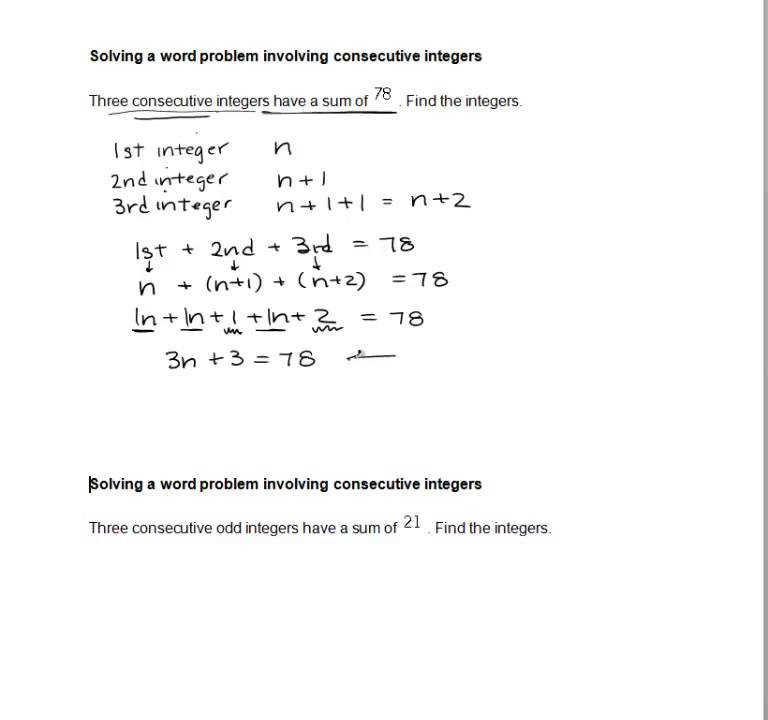## Consecutive Integer Word Problems Lesson

• Demonstrate an understanding of how to solve a Linear Equation in One Variable
• Learn the six-step process for solving any word problem that involves a Linear Equation in One Variable
• Learn how to check the solution for a word problem
• Learn how to set up and solve consecutive integer word problems## How to Solve a Consecutive Integer Word Problem

Six-step method for applications of linear equations in one variable.

• Write down the main objective of the problem
• If more than one unknown exists, we express the other unknowns in terms of this variable
• Write out an equation that describes the given situation
• Solve the equation
• State the answer using a nice clear sentence
• We need to make sure the answer is reasonable. In other words, if asked how many miles were driven to the store, the answer shouldn't be (-3) as we can't drive a negative amount of miles.

## Consecutive Integer Word Problems

• We are asked to find three consecutive integers.
• Let x = smallest consecutive integer
• Then x + 1 = the middle consecutive integer
• Then (x + 1) + 1 = the largest consecutive integer
• If we combine the three consecutive integers: x, (x + 1), (x + 1) + 1, our result is (equals) 21.
• x + (x + 1) + (x + 1) + 1 = 21
• x + x + 1 + x + 1 + 1 = 21
• 3x + 3 = 21
• 3x + 3 - 3 = 21 - 3
• 3x = 18
• 3/3 x = 18/3
• x = 6
• Since x represents the smallest consecutive integer, we know the smallest consecutive integer is 6.
• The middle consecutive integer is 7, from (6 + 1).
• The largest consecutive integer is 8, from (7 + 1).
• Our three consecutive integers are 6, 7, and 8.
• We know that the sum of the consecutive integers is 21.
• Check: 6 + 7 + 8 = 21
• 13 + 8 = 21
• 21 = 21
• Since our three consecutive integers sum to 21, our answer is correct.
• We are asked to find three consecutive odd integers.
• Consecutive odd integers differ by 2, (1, 3, 5, 7, 9,...)
• Let x = smallest consecutive odd integer
• Then x + 2 = the middle consecutive odd integer
• Then (x + 2) + 2 = the largest consecutive odd integer
• If we combine the three consecutive integers: x, (x + 2), (x + 2) + 2, our result is (equals) 57.
• x + (x + 2) + (x + 2) + 2 = 57
• x + x + 2 + x + 2 + 2 = 57
• 3x + 6 = 57
• 3x + 6 - 6 = 57 - 6
• 3x = 51
• 3/3 x = 51/3
• x = 17
• Since x represents the smallest consecutive odd integer, we know the smallest consecutive odd integer is 17.
• The middle consecutive odd integer is 19, from (17 + 2).
• The largest consecutive odd integer is 21, from (19 + 2).
• Our three consecutive odd integers are 17, 19, and 21.
• We know that the sum of the consecutive integers is 57.
• Check: 17 + 19 + 21 = 57
• 36 + 21 = 57
• 57 = 57
• Since our three consecutive integers sum to 57, our answer is correct.

## Skills Check:

Solve each word problem.

The sum of two pages that face each other in a book is 177. What are the page numbers?

The sum of three consecutive even integers is 102. Find the integers.

When the smaller of two consecutive integers is added to 3 times the larger, the result is -37. Find the two integers.

Congrats, Your Score is 100 %

Better Luck Next Time, Your Score is %

Ready for more? Watch the Step by Step Video Lesson   |   Take the Practice Test

## Algebra: Consecutive Integer Problems

In these lessons, we will learn how to solve

• consecutive integer word problems
• consecutive even integer word problems
• consecutive odd integer word problems

Related Pages Integers Word Problems Consecutive Integer Word Problems Consecutive Integers 2 Consecutive Even Integer Problems Consecutive Odd Integer Problems More Algebra Word Problems

## What Are Consecutive Integers?

Consecutive integers are integers that follow in sequence, each number being 1 more than the previous number, represented by n, n + 1, n + 2, n + 3, …, where n is any integer. For example: 23, 24, 25, …

If we start with an even number and each number in the sequence is 2 more than the previous number then we will get consecutive even integers . For example: 16,18, 20, …

If we start with an odd number and each number in the sequence is 2 more than the previous number then we will get consecutive odd integers . For example: 33, 35, 37, …

The following diagram shows an example of a consecutive integer problem. Scroll down the page for more examples and solutions on consecutive integer problems.Consecutive Integer Problems

Consecutive integer problems are word problems that involve consecutive integers .

The following are common examples of consecutive integer problems.

Example: The sum of the least and greatest of 3 consecutive integers is 60. What are the values of the 3 integers?

Solution: Step 1 : Assign variables: Let x = least integer x + 1 = middle integer x + 2 = greatest integer

Translate sentence into an equation. Sentence: The sum of the least and greatest is 60. Rewrite sentence: x + (x + 2) = 60

Step 2: Solve the equation Combine like terms 2x + 2 = 60

Step 3: Check your answer 29 + 29 + 2 = 60 The question wants all the 3 consecutive numbers: 29, 30 and 31

Answer: The 3 consecutive numbers are 29, 30 and 31.

Consecutive Odd Integers

Example 2: The lengths of the sides of a triangle are consecutive odd numbers. What is the length of the longest side if the perimeter is 45?

Solution: Step 1: Being consecutive odd numbers we need to add 2 to the previous number. Assign variables: Let x = length of shortest side x + 2 = length of medium side x + 4 = length of longest side

Step 2: Write out the formula for perimeter of triangle . P = sum of the three sides

Step 3: Plug in the values from the question and from the sketch. 45 = x + x + 2 + x + 4

Combine like terms 45 = 3x + 6

Isolate variable x 3x = 45 – 6 3x = 39 x =13

Step 3: Check your answer 13 + 13 + 2 + 13 + 4 = 45

Be careful! The question requires the length of the longest side. The length of longest = 13 + 4 =17

Answer: The length of longest side is 17

Consecutive Even Integers

Example 3: John has a board that is 5 feet long. He plans to use it to make 4 shelves whose lengths are to be a series of consecutive even numbers. How long should each shelf be in inches?

Solution: Step 1: Being consecutive even numbers we need to add 2 to the previous number. Assign variables: Let x = length of first shelf x + 2 = length of second shelf x + 4 = length of third shelf x + 6 = length of fourth shelf

Step 2: Convert 5 feet to inches 5 × 12 = 60

Step 3: Sum of the 4 shelves is 60 x + x + 2 + x + 4 + x + 6 = 60

Combine like terms 4x + 12 = 60

Isolate variable x 4x = 60 – 12 4x = 48 x = 12

Step 3: Check your answer 12 + 12 + 2 + 12 + 4 + 12 + 6 = 60

The lengths of the shelves should be 12, 14, 16 and 18.

Answer: The lengths of the shelves in inches should be 12, 14, 16 and 18.

How to find consecutive integers, consecutive odd integers, or consecutive even integers that add up to a given number

• The sum of three consecutive integers is 657; find the integers.
• The sum of two consecutive integers is 519; find the integers.
• The sum of three consecutive even integers is 528; find the integers.
• The sum of three consecutive odd integers is 597; find the integers.

The following video shows how to solve the integer word problems.

• The sum of two consecutive integers is 99. Find the value of the smaller integer.
• The sum of two consecutive odd integers is 40. What are the integers?
• The sum of three consecutive even integers is 30. Find the integers.

How to solve consecutive integer word problems?

Example: The sum of three consecutive integers is 24. Find the integers.If you're seeing this message, it means we're having trouble loading external resources on our website.

If you're behind a web filter, please make sure that the domains *.kastatic.org and *.kasandbox.org are unblocked.

## Course: 8th grade   >   Unit 2

Sums of consecutive integers.

• Sum of integers challenge
• Solving equations with one unknown: FAQ## Want to join the conversation?

• Upvote Button navigates to signup page
• Downvote Button navigates to signup page
• Flag Button navigates to signup page## SOLVING WORD PROBLEMS INVOLVING CONSECUTIVE INTEGERS

Consecutive numbers are numbers that follow each other in order. They have a difference of 1 between every two numbers.

To solve word problems regarding consecutive integers, it is important to note :

Consecutive Integers :

If the first integer be x, then three consecutive integers are

x, x + 1, x + 2

Consecutive Even Integers :

If the first even integer be x, then three consecutive integers are

x, x + 2, x + 4

Consecutive Odd Integers :

If the first odd integer be x, then three consecutive integers are

Problem 1 :

If the average of three consecutive integers is 26, find the integers.

Let x , ( x + 1) and (x + 2) be the three consecutive integers.

Average of three consecutive integers = 26

Multiply both sides by 3.

3x + 3 = 78

Subtract 3 from both sides.

Divide both sides by 3.

Therefore, the three consecutive integers are 25, 26 and 27.

Problem 2 :

If the average of three consecutive even integers is 14, find the integers.

Let x , ( x + 2) and (x + 4) be the three consecutive even integers.

Average of three consecutive even integers = 14

3x + 6 = 42

Subtract 6 from both sides.

Therefore, the three consecutive even integers are 12, 14 and 16.

Problem 3 :

Among the three consecutive odd integers,  26 less than thrice the largest integer is equal to  twice the smallest integer. Find the integers.

Let x , ( x + 2) and (x + 4) be the three consecutive odd integers.

3(x + 4) - 25 = 2x

3x + 12 - 25 = 2x

3x - 13 = 2x

Therefore, the three consecutive odd integers are 12, 14 and 16.

Problem 4 :

Find two consecutive natural numbers whose product is 30.

Let x , ( x + 1) be the two consecutive integers.

Product of two consecutive integers = 30

x(x + 1) = 30

x 2  + x = 30

x 2  + x - 30 = 0

x 2  - 5x + 6x - 30 = 0

x(x - 5) + 6(x - 5) = 0

(x - 5)(x - 6) = 0

x - 5 = 0  or  x + 6 = 0

x = 5  or  x = -6

Since the natural numbers are always positive, x can not be -6.

Therefore, the required two consecutive natural numbers are 5 and 6.

Verification :

Product of two positive integer is 30.

5(6) = 30

30 = 30

Problem 5 :

There are three consecutive positive integers such that the sum of the square of first and the product of the other two is 154. Find the integers.

Let x , ( x + 1) and ( x + 2) be the required three consecutive integers

The sum of the squares of first and the product of the other two is 154.

x 2  + (x + 1)(x + 2) = 154

x 2  + x 2  + 2x + x + 2 = 154

2x 2  + 3x + 2 = 154

2x 2  + 3x + 2 - 154 = 0

2x 2  + 3x - 152 = 0

2x 2  - 16x + 19x - 152 = 0

2x(x - 8) + 19(x - 8) = 0

(x - 8)(2x + 19) = 0

x - 8 = 0  or  2x + 19 = 0

x = 8  or  x = -9.5

Since the numbers are positive integers, x can not be -9.5.

Therefore, the required three consecutive positive integers are 8, 9 and 10.

The sum of the square of first and the product of the other two is 154.

8 2  + (9) (10) = 154

64 + 90 = 154

154 = 154

Kindly mail your feedback to   [email protected]

• Sat Math Practice
• SAT Math Worksheets
• PEMDAS Rule
• BODMAS rule
• GEMDAS Order of Operations
• Math Calculators
• Transformations of Functions
• Order of rotational symmetry
• Lines of symmetry
• Compound Angles
• Quantitative Aptitude Tricks
• Trigonometric ratio table
• Word Problems
• Times Table Shortcuts
• 10th CBSE solution
• PSAT Math Preparation
• Laws of Exponents

## Recent Articles## SAT Math Questions and Answers (No Calculator)

Dec 01, 23 08:59 PM

## Linear Equations in One Variable

Dec 01, 23 11:01 AM

## Mixture Problems Worksheet

Nov 30, 23 04:36 AM#### IMAGES

1. Consecutive Integer Word Problems 32. PPT3. Solving Integer Word Problems4. Solving a word problem involving consecutive integers5. an info sheet with the words, which are in different colors and font options for students to use6. Consecutive Integer Word Problems#### VIDEO

1. Consecutive Number Word Problems

2. integers word problem part 1 cbse 6th @mathworldtamil #shortvideo

3. Integers PROBLEMS Word Problems Business and general MATHEMATICS part 3

4. integers word problem part 2 @mathworldtamil #shortvideo

5. Addition and Subtraction Word Problem on Integers

6. Word problems related to integers || Word Problem Series || Lesson 1

1. What Are the Six Steps of Problem Solving?

The six steps of problem solving involve problem definition, problem analysis, developing possible solutions, selecting a solution, implementing the solution and evaluating the outcome. Problem solving models are used to address issues that...

2. How Can You Find Consecutive Odd Numbers?

Consecutive odd numbers are any two odd numbers with only one even number between them. To find a pair of consecutive odd numbers when given their sum, set up the equation 2x + 2 to equal the sum. Solve it for the variable “x.”

3. Solve for Y in Terms of X?

According to the University of Regina, another way to express solving for y in terms of x is solving an equation for y. The solution is not a numerical value; instead, it is an expression equal to y involving the variable x. An example prob...

4. Solving a word problem involving consecutive integers

MAT 0028 ALEKS Module 4 - Solving a word problem involving consecutive integers.

5. Solving a word problem involving consecutive integers

For a complete list of Timely Math Tutor videos by course: www.timelymathtutor.com.

6. Consecutive Integer Word Problems Lesson

Consecutive Integer Word Problems · Read the problem carefully and determine what you are asked to find · Assign a variable to represent the unknown · Write out an

7. Consecutive Integers Word Problems

This algebra lesson math video tutorial explains how to solve consecutive integers word problems. It explains how to do it the simple way.

8. Sum of Consecutive Integers Word Problems

Examples of Solving the Sum of Consecutive Integers · We need to ADD three integers that are consecutive · The numbers are one unit apart from each other · Each

9. Algebra: Consecutive Integer Problems

Consecutive Integer Problems - word problems involving consecutive integers, consecutive even and odd integer word problems, how to solve algebra word

10. Applied (Word) Problems NoteSheet Consecutive Integers Examples

of 7 times the smallest and twice the largest is -91 . Solving Word Problems: Solving Problems Involving

11. Q: How to do consecutive integer word problems?

... integer as x + 1. Set up an equation based on the information given in the problem. This equation will involve the variables you defined in step 2. Solve

12. Sums of consecutive integers (video)

Then, apply the math described in the word problem to set up an equation. Sal's

13. Solving Word Problems Involving Consecutive Integers

SOLVING WORD PROBLEMS INVOLVING CONSECUTIVE INTEGERS · Consecutive numbers are numbers that follow each other in order. · To solve word problems regarding

14. Word Problems: Consecutive Integer Problems

Word Problems: Consecutive Integer Problems. Jefferson Davis Learning Center, Sandra Peterson. Solve each problem below. Answers. 1. Find two consecutive